MORE IN Circuits and Transmission Lines
MU Electronics and Telecom Engineering (Semester 3)
Circuits and Transmission Lines
December 2012
Total marks: --
Total time: --
INSTRUCTIONS
(1) Assume appropriate data and state your reasons
(2) Marks are given to the right of every question
(3) Draw neat diagrams wherever necessary

1 (a) For maximum power transfer find the value of ZL if
(i) ZL is impedance
(ii) ZL is pure resistance :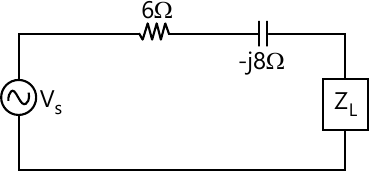5 M
1 (b) find initial value of, f(t)= 20-10t-e-25t
verify using initial value theorem.
5 M
1 (c) In the network given below, initial value of IL =4A and VC=100. Find IC(0+):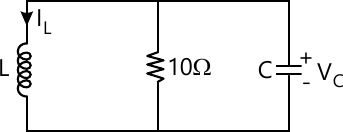5 M
1 (d) Current I1 and I2 entering at 1 and port 2 respectively of two port network are given by following equation.
I1 =0.5V1 - 0.2V2
I2 = -0.2V1 +V2
Obtain T and Π(pi) representation.
5 M
Attempt any FOUR from the following
1 (e) For network shown, find VC /V and draw pole-zero plot.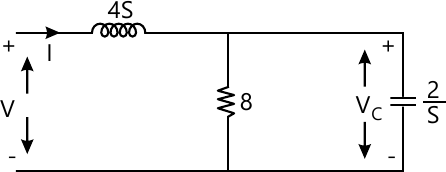5 M

2 (a) Using mesh analysis find power supplied by the dependent source.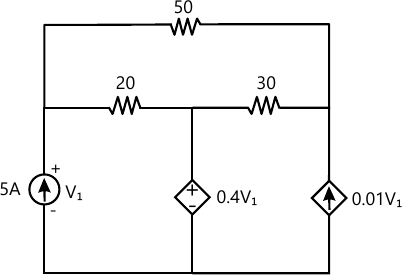10 M
2 (b) Find current supplied by source.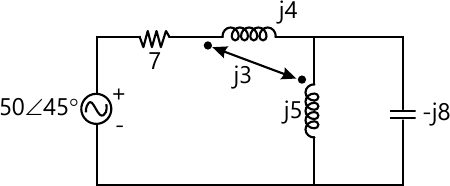10 M

3 (a) Write B and Q matrix for the Graph shown.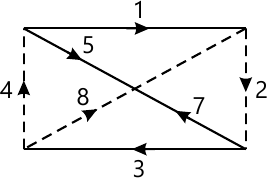10 M
3 (b) Draw Bode plot for the function G(s). Find gain margin, phase margin and comment on stability.
$G(s)= \frac{2(s+0.25)}{s^2(s+1)(s+0.5)}$
10 M

4 (a) Switch is opened at t=0 with initial conditions as shown. Find
$v_1, \frac{dv_1}{dt}, \frac{dv_2}{dt}\ at \ time\ 0^+$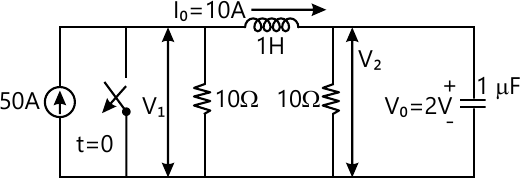10 M
4 (b) Find Y parameter using interconnection: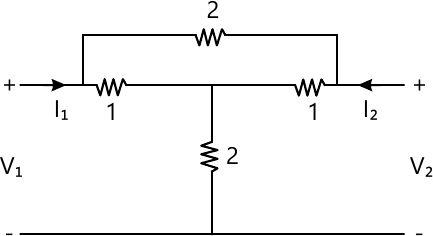10 M

5 (a) In the network key is closed at t=0. Find i1 (0+), i2(0+ and i3 (0+).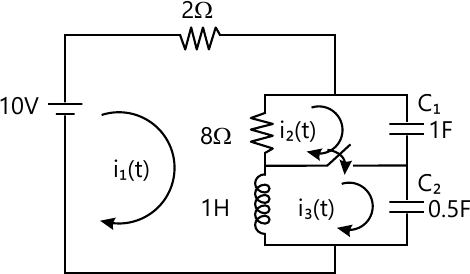10 M
5 (b) Find i(t).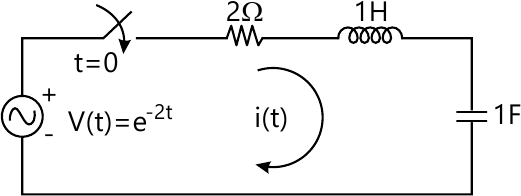10 M

6 (a) The circuit attain steady state with switch at position (a) & is moved to position (b) at t=0. Find V(t) for t ≥ 0.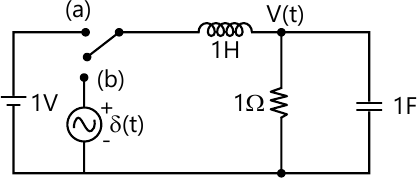10 M
6 (b) Find Z11, Z21 and G21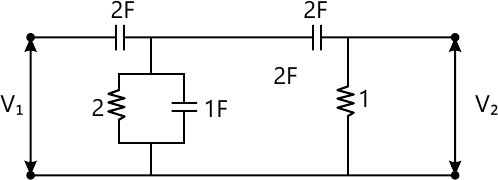10 M

7 (a) Realize following functon in Foster II form
$Z(s)=\frac{(s^2+1)(s^2+3)}{s(s^2+2)(s^2+4)}$
10 M
7 (b) Check following polynomials for Hurtwitz -
$\ \left(i\right)\ \ p\left(s\right)=S^4+4s^2+8$
$\left(ii\right)\ \ p\left(s\right)=s^4+s^3+5s^2+3s+4$
10 M

More question papers from Circuits and Transmission Lines